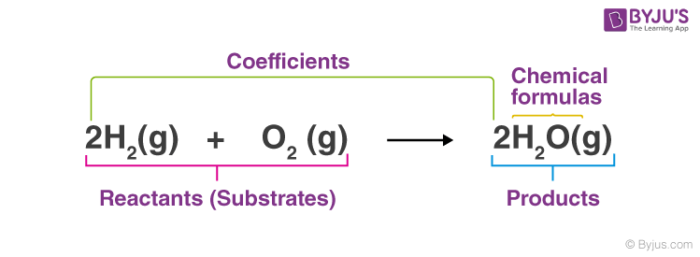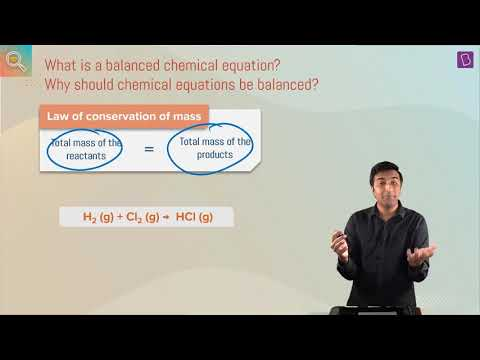# Chemical Equations

Chemical equations are symbolic representations of chemical reactions in which the reactants and the products are expressed in terms of their respective chemical formulae.

## What is a Chemical Equation?

Chemical equations make use of symbols to represent factors such as the direction of the reaction and the physical states of the reacting entities. Chemical equations were first formulated by the French chemist Jean Beguin in the year 1615.

Chemical reactions can be represented on paper with the help of chemical equations, an example for which is represented below (for the reaction between hydrogen gas and oxygen gas to form water).

2H2 + O2 → 2H2O

It can be observed in the example provided above that the reacting entities are written on the left-hand side whereas the products that are formed from the chemical reactions are written on the right-hand side of the chemical equation.It can also be observed that there are coefficients assigned to each of the symbols of the corresponding reactants and products. These coefficients of entities in a chemical equation are the exact value of the stoichiometric number for that entity.

### Representing the Direction of the Chemical Reaction

The reactants and the products (for which the chemical formulae are written in chemical equations) can be separated by one of the following four symbols.

• In order to describe a net forward reaction, the symbol ‘→’ is used.
• In order to describe a state of chemical equilibrium, the symbol ‘⇌’ is used.
• To denote stoichiometric relationships, the ‘=’ symbol is used.
• In order to describe a reaction that occurs in both forward and backward directions, the symbol ‘⇄’ is used.

Multiple entities on either side of the reaction symbols described above are separated from each other with the help of the ‘+’ symbol in a chemical equation. It can be noted that the ‘→’ symbol, when used in a chemical equation, is often read as ‘gives rise to’ or ‘yields’.

### Representing the Physical States of the Reacting Entities

Apart from the stoichiometric coefficients of the reacting and the produced entities, symbols enclosed in parentheses are also written adjacent to them in order to describe their physical states over the course of the chemical reaction. These symbols may be one of the following.

• The symbol (s) describes an entity in the solid state
• The symbol (l) denotes the liquid state of an entity
• The symbol (g) implies that the entity is in the gaseous state.
• The (aq) symbol corresponding to an entity in a chemical equation denotes an aqueous solution of that entity.

In some reactions, a reactant or a product may be in the form of a precipitate which is insoluble in the solution that the reaction is taking place in. The ‘↓’ symbol is written next to the chemical formula of these entities to describe them as precipitates.

### How is the Input of Energy Represented in a Chemical Equation?

Some chemical reactions require an input of energy in order to proceed. The energy requirements of these reactions are described above the arrow symbol (forward reaction) in their corresponding chemical equations with the help of the following symbols

• The Greek letter delta in its capitalized form (Δ) is used to state that an input of heat energy is required by the reaction.
• The formula ‘hv’ which describes the energy of a photon is used above the arrow symbol to state that the reaction requires an input of light to proceed.

It is important to note that the stoichiometric coefficients that are assigned to each entity in the chemical equation are used to make the entire equation obey the law of conservation of charge and the law of conservation of mass.

## Ionic Chemical Equations

In ionic chemical equations, the electrolytes (substances that dissociate into ions when dissolved in polar solvents) are split up and written as separate ions. These equations are very useful in describing single displacement reactions and salt metathesis reactions (generally referred to as double displacement reactions).

### Example

An example of an ionic chemical equation is provided below.

Chemical Equation: CaCl2 + 2AgNO3 → Ca(NO3)2 + 2AgCl↓

Ionic Equation: Ca2+ + 2Cl + 2Ag+ + 2NO3 → Ca2+ + 2NO3 + 2AgCl↓

Comparing the reactants and the products of the ionic equation and the chemical equation, it can be observed that the Ca2+ (calcium ion) and the NO3 (nitrate) ions are present on both sides of the ionic equation. These ions are referred to as spectator ions because they do not participate in the chemical reaction.

The net ionic equation for the example above can be written by removing the spectator ions and writing only the reaction between the participating ions, as shown below.

2Cl + 2Ag+ → 2AgCl↓

This ionic chemical equation can be interpreted as follows – two chloride ions originating from calcium chloride react with two silver cations originating from silver nitrate, forming a precipitate of silver chloride as the product.

## Recommended Videos

#### Chemical Reactions and Equations Balancing of a Chemical Reaction#### Balancing A Chemical Equation## Frequently Asked Questions – FAQs

### What are Chemical Equations?

They are equations that make use of chemical formulae and symbols to represent chemical reactions. The left-hand side of a chemical equation represents the reactants and the right-hand side represents the products. These entities are separated by a symbol that describes the direction of the reaction. Each reacting entity is also assigned its corresponding stoichiometric coefficient.

### What are the Four Symbols used to Denote Physical States in these Equations?

The symbols used to denote the physical states of the reacting entities include:

(s) for solid.
(l) for liquid.
(g) for gas.
The aqueous solution of a chemical is often represented with the symbol (aq).

### What is an Ionic Equation?

The chemical equations in which electrolytes are represented in the form of dissociated ions are commonly referred to as ionic equations. They are often used to represent the displacement reactions that take place in aqueous mediums. In these reactions, some ions participate in the reaction and some do not. The ions that do not react are called spectator ions and are usually omitted from the net ionic equation.

### List some Examples of Chemical Equations.

A few examples of chemical equations are listed in bulleted text below.

PCl5 + 4H2O → H3PO4 + 5HCl
SnO2 + 2H2 → 2H2O + Sn
TiCl4 + 2H2O → TiO2 + 4HCl
H3PO4 + 3KOH → K3PO4 + 3H2O
Na2S + 2AgI → 2NaI + Ag2S

### How do you write chemical formulas?

How do you write chemical formulas?
There’s a particular way of writing what’s in a molecule called a chemical formula. The chemical formulae for all the elements that form each molecule and uses a small number to the bottom right of an element’s symbol to stand for the number of atoms of that element. For example, the chemical formula for water is H2O.

Thus, the fundamentals of writing chemical and ionic equations are discussed. To learn more about chemical equations and how to balance them with the correct stoichiometric coefficients, register with BYJU’S and download the mobile application on your smartphone.

Test your Knowledge on Chemical equation!